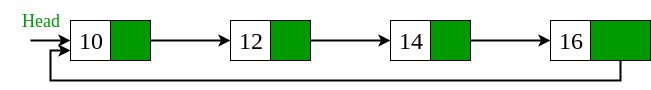• Difficulty Level : Easy
• Last Updated : 07 Jul, 2021

Given a singly linked list, find if the linked list is circular or not. A linked list is called circular if it is not NULL-terminated and all nodes are connected in the form of a cycle. Below is an example of a circular linked list.An empty linked list is considered as circular.
Note that this problem is different from cycle detection problem, here all nodes have to be part of cycle.

The idea is to store head of the linked list and traverse it. If we reach NULL, linked list is not circular. If reach head again, linked list is circular.

## C++

 `// C++ program to check if linked list is circular``#include``using` `namespace` `std;` `/* Link list Node */``struct` `Node``{``    ``int` `data;``    ``struct` `Node* next;``};` `/* This function returns true if given linked``   ``list is circular, else false. */``bool` `isCircular(``struct` `Node *head)``{``    ``// An empty linked list is circular``    ``if` `(head == NULL)``       ``return` `true``;` `    ``// Next of head``    ``struct` `Node *node = head->next;` `    ``// This loop would stop in both cases (1) If``    ``// Circular (2) Not circular``    ``while` `(node != NULL && node != head)``       ``node = node->next;` `    ``// If loop stopped because of circular``    ``// condition``    ``return` `(node == head);``}` `// Utility function to create a new node.``Node *newNode(``int` `data)``{``    ``struct` `Node *temp = ``new` `Node;``    ``temp->data = data;``    ``temp->next = NULL;``    ``return` `temp;``}` `/* Driver program to test above function*/``int` `main()``{``    ``/* Start with the empty list */``    ``struct` `Node* head = newNode(1);``    ``head->next = newNode(2);``    ``head->next->next = newNode(3);``    ``head->next->next->next = newNode(4);` `    ``isCircular(head)? cout << ``"Yes\n"` `:``                      ``cout << ``"No\n"` `;` `    ``// Making linked list circular``    ``head->next->next->next->next = head;` `    ``isCircular(head)? cout << ``"Yes\n"` `:``                      ``cout << ``"No\n"` `;` `    ``return` `0;``}`

## Java

 `// Java program to check if``// linked list is circular``import` `java.util.*;` `class` `GFG``{``    ` `/* Link list Node */``static` `class` `Node``{``    ``int` `data;``    ``Node next;``}` `/*This function returns true if given linked``list is circular, else false. */``static` `boolean` `isCircular( Node head)``{``    ``// An empty linked list is circular``    ``if` `(head == ``null``)``    ``return` `true``;` `    ``// Next of head``    ``Node node = head.next;` `    ``// This loop would stop in both cases (1) If``    ``// Circular (2) Not circular``    ``while` `(node != ``null` `&& node != head)``    ``node = node.next;` `    ``// If loop stopped because of circular``    ``// condition``    ``return` `(node == head);``}` `// Utility function to create a new node.``static` `Node newNode(``int` `data)``{``    ``Node temp = ``new` `Node();``    ``temp.data = data;``    ``temp.next = ``null``;``    ``return` `temp;``}` `/* Driver code*/``public` `static` `void` `main(String args[])``{``    ``/* Start with the empty list */``    ``Node head = newNode(``1``);``    ``head.next = newNode(``2``);``    ``head.next.next = newNode(``3``);``    ``head.next.next.next = newNode(``4``);` `    ``System.out.print(isCircular(head)? ``"Yes\n"` `:``                    ``"No\n"` `);` `    ``// Making linked list circular``    ``head.next.next.next.next = head;` `    ``System.out.print(isCircular(head)? ``"Yes\n"` `:``                    ``"No\n"` `);` `}``}` `// This code contributed by Arnab Kundu`

## Python3

 `# A simple Python program to check if a linked list is circular` `# Node class``class` `Node:` `    ``# Function to initialise the node object``    ``def` `__init__(``self``, data):``        ``self``.data ``=` `data ``# Assign data``        ``self``.``next` `=` `None` `# Initialize next as null`  `# Linked List class contains a Node object``class` `LinkedList:` `    ``# Function to initialize head``    ``def` `__init__(``self``):``        ``self``.head ``=` `None` `def` `Circular(head):``    ``if` `head``=``=``None``:``        ``return` `True``        ` `    ``# Next of head``    ``node ``=` `head.``next``    ``i ``=` `0``    ` `    ``# This loop would stop in both cases (1) If``    ``# Circular (2) Not circular``    ``while``((node ``is` `not` `None``) ``and` `(node ``is` `not` `head)):``        ``i ``=` `i ``+` `1``        ``node ``=` `node.``next``    ` `    ``return``(node``=``=``head)`  `# Code execution starts here``if` `__name__``=``=``'__main__'``:``    ``llist ``=` `LinkedList()``    ``llist.head ``=` `Node(``1``)``    ``second ``=` `Node(``2``)``    ``third ``=` `Node(``3``)``    ``fourth ``=` `Node(``4``)``    ` `    ``llist.head.``next` `=` `second;``    ``second.``next` `=` `third;``    ``third.``next` `=` `fourth``    ` `    ``if` `(Circular(llist.head)):``        ``print``(``'Yes'``)``    ``else``:``        ``print``(``'No'``)``    ` `    ``fourth.``next` `=` `llist.head``    ` `    ``if` `(Circular(llist.head)):``        ``print``(``'Yes'``)``    ``else``:``        ``print``(``'No'``)``        ` `# This code is contributed by Sanket Badhe`

## C#

 `// C# program to check if``// linked list is circular``using` `System;``public` `class` `GFG``{``    ` `/* Link list Node */``public` `class` `Node``{``    ``public` `int` `data;``    ``public` `Node next;``}` `/*This function returns true if given linked``list is circular, else false. */``static` `bool` `isCircular( Node head)``{``    ``// An empty linked list is circular``    ``if` `(head == ``null``)``    ``return` `true``;` `    ``// Next of head``    ``Node node = head.next;` `    ``// This loop would stop in both cases (1) If``    ``// Circular (2) Not circular``    ``while` `(node != ``null` `&& node != head)``    ``node = node.next;` `    ``// If loop stopped because of circular``    ``// condition``    ``return` `(node == head);``}` `// Utility function to create a new node.``static` `Node newNode(``int` `data)``{``    ``Node temp = ``new` `Node();``    ``temp.data = data;``    ``temp.next = ``null``;``    ``return` `temp;``}` `/* Driver code*/``public` `static` `void` `Main(String []args)``{``    ``/* Start with the empty list */``    ``Node head = newNode(1);``    ``head.next = newNode(2);``    ``head.next.next = newNode(3);``    ``head.next.next.next = newNode(4);` `    ``Console.Write(isCircular(head)? ``"Yes\n"` `:``                    ``"No\n"` `);` `    ``// Making linked list circular``    ``head.next.next.next.next = head;` `    ``Console.Write(isCircular(head)? ``"Yes\n"` `:``                    ``"No\n"` `);` `}``}``// This code has been contributed by 29AjayKumar`

## Javascript

 ``

Output :

```No
Yes```

?list=PLqM7alHXFySH41ZxzrPNj2pAYPOI8ITe7
This article is contributed by Shivam Gupta. If you like GeeksforGeeks and would like to contribute, you can also write an article and mail your article to review-team@geeksforgeeks.org. See your article appearing on the GeeksforGeeks main page and help other Geeks.